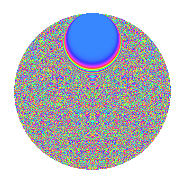# Properties

 Label 1849.2.mLevel 1849 Weight 2 Character orbit m Rep. character $$\chi_{1849}(4,\cdot)$$ Character field $$\Q(\zeta_{301})$$ Dimension 39312 Sturm bound 315

# Related objects

## Defining parameters

 Level: $$N$$ $$=$$ $$1849 = 43^{2}$$ Weight: $$k$$ $$=$$ $$2$$ Character orbit: $$[\chi]$$ $$=$$ 1849.m (of order $$301$$ and degree $$252$$) Character conductor: $$\operatorname{cond}(\chi)$$ $$=$$ $$1849$$ Character field: $$\Q(\zeta_{301})$$ Sturm bound: $$315$$

## Dimensions

The following table gives the dimensions of various subspaces of $$M_{2}(1849, [\chi])$$.

Total New Old
Modular forms 39816 39816 0
Cusp forms 39312 39312 0
Eisenstein series 504 504 0

## Trace form

 $$39312q - 256q^{2} - 257q^{3} - 102q^{4} - 257q^{5} - 256q^{6} - 242q^{7} - 252q^{8} - 97q^{9} + O(q^{10})$$ $$39312q - 256q^{2} - 257q^{3} - 102q^{4} - 257q^{5} - 256q^{6} - 242q^{7} - 252q^{8} - 97q^{9} - 265q^{10} - 261q^{11} - 269q^{12} - 253q^{13} - 200q^{14} - 249q^{15} - 112q^{16} - 261q^{17} - 271q^{18} - 41q^{19} - 273q^{20} - 266q^{21} - 264q^{22} - 173q^{23} - 254q^{24} - 101q^{25} - 261q^{26} - 287q^{27} - 279q^{28} - 273q^{29} + 59q^{30} - 147q^{31} - 222q^{32} - 278q^{33} - 270q^{34} - 90q^{35} - 1166q^{36} - 240q^{37} - 145q^{38} - 263q^{39} - 277q^{40} - 282q^{41} - 220q^{42} - 216q^{43} - 220q^{44} - 232q^{45} - 274q^{46} - 272q^{47} - 269q^{48} - 1178q^{49} - 270q^{50} - 234q^{51} - 257q^{52} - 159q^{53} - 286q^{54} - 277q^{55} - 253q^{56} - 120q^{57} - 233q^{58} - 288q^{59} - 277q^{60} - 289q^{61} - 303q^{62} - 267q^{63} - 132q^{64} - 277q^{65} + 937q^{66} - 235q^{67} + 299q^{68} - 259q^{69} - 232q^{70} - 312q^{71} + 373q^{72} - 270q^{73} - 242q^{74} + 376q^{75} - 118q^{76} - 237q^{77} - 239q^{78} - 268q^{79} - 220q^{80} - 22q^{81} + 261q^{82} - 238q^{83} - 230q^{84} - 230q^{85} - 210q^{86} + 318q^{87} - 230q^{88} - 280q^{89} + 528q^{90} - 254q^{91} - 270q^{92} - 230q^{93} + 281q^{94} - 26q^{95} - 274q^{96} + 297q^{97} - 284q^{98} - 42q^{99} + O(q^{100})$$

## Decomposition of $$S_{2}^{\mathrm{new}}(1849, [\chi])$$ into newform subspaces

The newforms in this space have not yet been added to the LMFDB.

## Hecke characteristic polynomials

There are no characteristic polynomials of Hecke operators in the database